### Would you like to switch to our mobile app?

Chapter 25 — Technical Indicators
Font type: Sans-Serif
Font size: Large

## Technical Indicators

Technical indicators are used in the same way as technical price patterns. Their purpose is to help you determine the future direction of a stock price, but they are slightly more complex than following price trends.

Momentum Indicator

The momentum indicator follows a very simple equation. It is the difference between the current market price of a share and the price of the same share from a certain number of days ago. The equation looks like this:

MI = (current price – price from N days ago)

The more often you trade, the shorter time interval you will want to use. If you are a longer-term trader, you will want to use a longer time interval. In this example, the interval is 7 days.The way to interpret these numbers is to plot them on a graph and follow this simple rule: When the momentum indicator becomes negative, that is a sign to sell. When the momentum indicator becomes positive, that is a sign to buy. In other words, you are buying when the stock is gaining momentum and selling when the stock is losing momentum. You might miss the initial reversal, but this method should still allow you to capture some gains.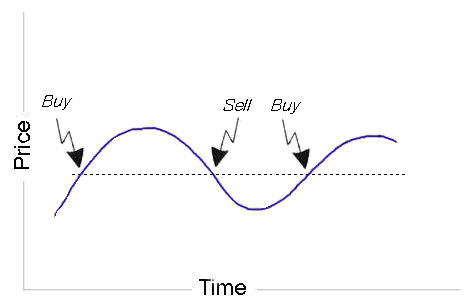Momentum Oscillator

The momentum oscillator is a normalized version of the indicator. It changes the values of the momentum indicator so that they will each fall between 1 and -1. This is done by first calculating the momentum indicator for a relatively long period of time, and then finding the largest number (smallest negative or largest positive). Divide all MI numbers by this baseline, and you will come up with the momentum oscillator numbers.

From the previous example, the highest MI number was 0.44. Diving all MI numbers by 0.44 brings up the new MO numbers.Extending this example to a longer time period and plotting the MO numbers on a chart will give you the same shape as the MI, but the graph will range between 1 and -1 and the buy and sell signals will come earlier. A good rule of thumb for the MO is to buy and sell when the numbers reach 0.80 and -0.80.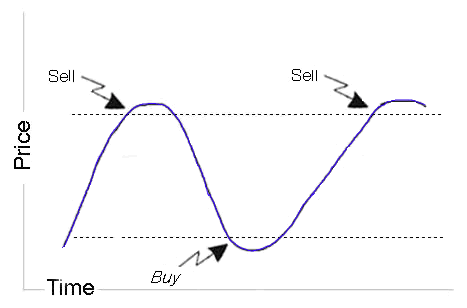The rate of Change Indicator

The purpose of the rate of change indicator is to show how quickly the price of a share is moving. The idea behind this indicator is the belief that if the price is moving up very quickly, it will soon be overbought and ready for a tumble. If the price is moving downward very quickly, it will soon be oversold.

The rate of change indicator is calculated by:

ROC = 100 – 100 / (1+X)

Where X = (average of all price rises during time period/average of all price declines during the time period)

The time period that you use is your choice and depends on your trading time frame. This indicator will fluctuate between 0 and 100, with 50 being considered neutral. When the ROC moves above 75, it is considered overbought (a sell signal). When it moves below 25, the stock is considered oversold (a buy signal).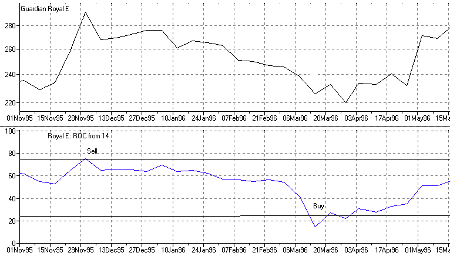Price Minus Average

The price minus average graph is simply a reconstruction of the moving average indicator. When you overlay a moving average on top of a normal price graph, sometimes the average will be above the price and sometimes it will be below the price. This indicator is simply the difference between the price and the average, making it easier to spot a buying opportunity. When the price minus average indicator moves from negative to positive, it is a buy signal. When it moves down below zero, it is a sell signal.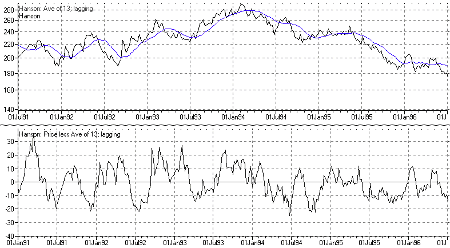Relative Strength Indicator

The relative strength indicator compares the current price of a stock with past prices. The formula is relatively complex and not worth calculating by hand, but the basic idea is that if a stock closes near its daily high it will tend to rise, and if a stock closes near its daily low, it will tend to fall.

The way to interpret an RSI graph is to consider any numbers above 70 to be an overbought stock that you should consider selling. Any number below 30 means that the stock is oversold and you should consider buying. All RSI numbers will be between 0 and 100, and the RSI indicator tends to be more reliable with very volatile stocks.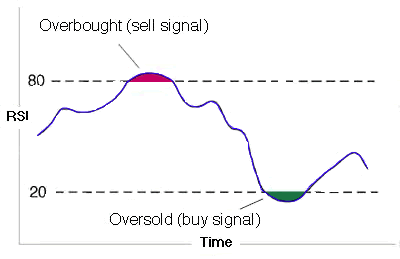Conclusion

This group of advanced technical charts and technical indicators should get you started if you wish to pursue a technical analysis based trading strategy. You should now be able to see buy and sell signals for different types of technical patterns, know what level of volume is associated with those patterns, and understand how to use a few different types of technical indicators. In most cases, your trading software will be able to show you the technical indicators without much effort, but you will likely need to search for patterns on your own. How you use this information is entirely up to you.